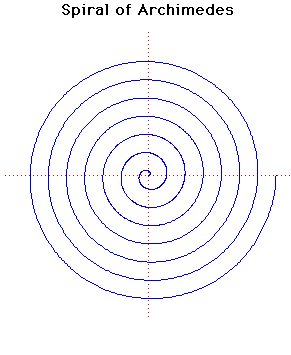# Spiral of ArchimedesPolar equation:
r = aθ

Click below to see one of the Associated curves.

Click THIS LINK to experiment interactively with this curve and its associated curves.

This spiral was studied by Archimedes in about 225 BC in a work On Spirals. It had already been considered by his friend Conon.

Archimedes was able to work out the lengths of various tangents to the spiral. It can be used to trisect an angle and square the circle.

The curve can be used as a cam to convert uniform angular motion into uniform linear motion. The cam consists of one arch of the spiral above the x-axis together with its reflection in the x-axis. Rotating this with uniform angular velocity about its centre will result in uniform linear motion of the point where it crosses the y-axis.

Taking the pole as the centre of inversion, the spiral of Archimedes r = aθ inverts to the hyperbolic spiral r = a/θ.

JOC/EFR/BS January 1997